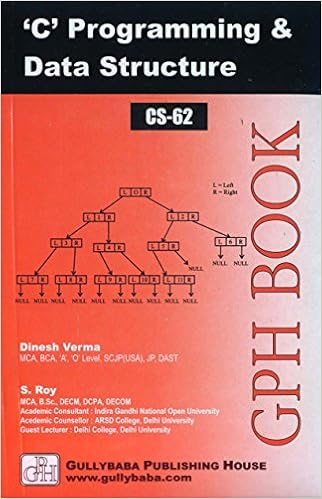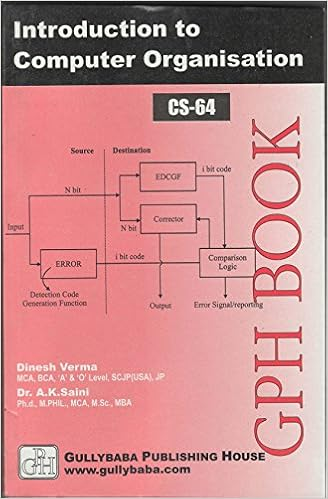IGNOU BCA MCA.comIGNOU Assignment mca CS-02-TMA January 2007

CS-02: Introduction to Software
Semester: I
Assignment Type : TMA
Last date: 15th April, 2007

This is a Tutor Marked Assignment. There are five questions in this assignment. Answer all the questions. You may use illustrations and diagrams to enhance explanations.

Question 1: Write an algorithm / program that accepts a decimal number as input and convert to its hexadecimal equivalent. [marks: 0]

Question 2: Consider the following set of processes that arrived in the ready queue at the same time:

 Process CPU time P1 5 P2 3 P3 1 P4 7 P5 2
Consider the following scheduling algorithms: First Come First Serve (FCFS), SJF (shortest job first) and Round Robin (quantum = 1)
1. What is the turnaround time of each process for each of the above scheduling algorithms?
2. What is the waiting time of each process for each of the above scheduling algorithms?
[marks: 0]

Question 3: With reference to any of the multi-user and multitasking operating system, explain the features, advantages and limitations. [marks: 0]